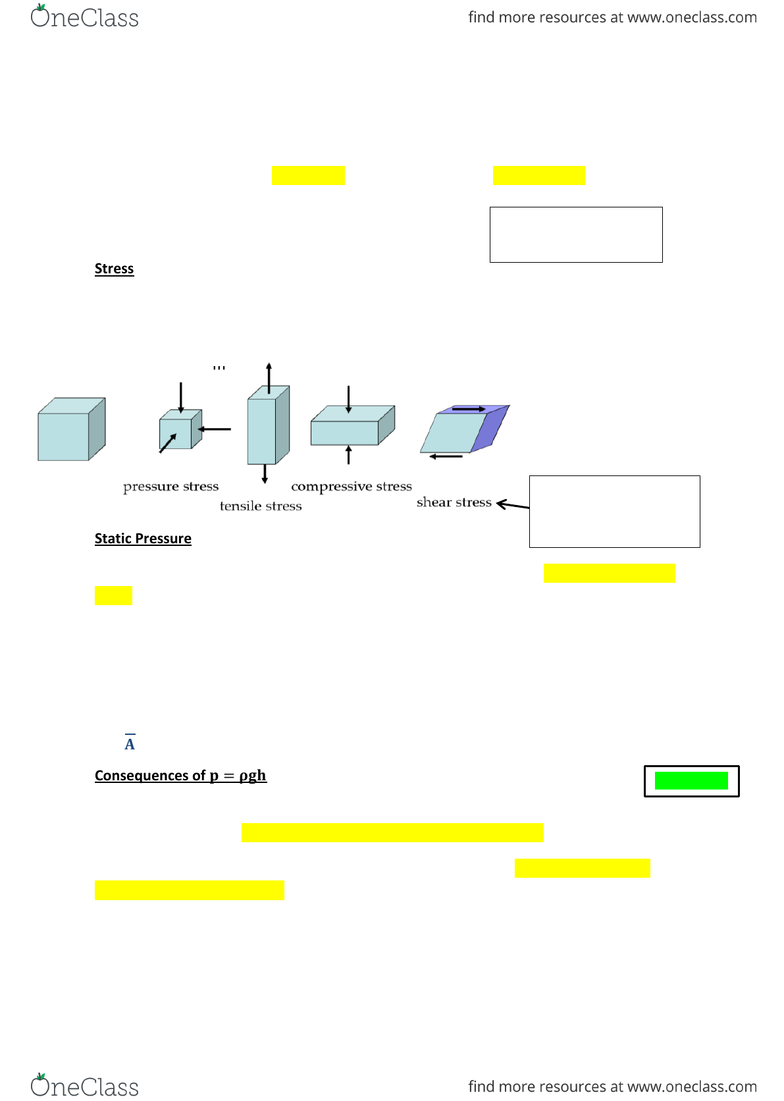Get 2 days of unlimited access
Class Notes (1,000,000)
NZ (300)
MU (20)
(6)
Lecture

# 280 .272 Lecture Notes - Net Force, Fluid Mechanics, Statics

Department
280
Course Code
280 .272
Professor
Patrick Janssen

Page:
of 4280.272 Notes
1
Can apply stress prior to
static conditions
Problem 1.1
Fluid Statics
Fluids are liquids or gases
Fluid mechanics consists of fluid statics (stationary fluids) and fluid dynamics (moving fluids)
The characteristic property of a fluid is that it is unable to
support a shear stress in static equilibrium
Stress
Defined as force per unit area and is measured in Pa
The force can be applied
in many directions
Static Pressure
In static equilibrium there is no shear stress so therefore there is only hydrostatic pressure
stress, which is a scalar.
Hydrostatic Pressure follows Pascal’s Law: Pressure is transmitted without loss through the
fluid system
Pressure at the Base:
 
 
Consequences of 
The static pressure will only be dependent upon the height. Therefore if the liquids are the
same density then the pressure will be the same at any horizontal line.
The pressure also increases in direct proportion to the depth. i.e. the deeper down the
liquid the higher the pressure.
Shear stress is two forces
moving relative to
another
280.272 Notes
2
Problem 1.2
Problem 1.3
Problem 1.4
Gauge Pressure
Defined as the difference between measured pressure and the instrument pressure
NB: in problems this normally relates to setting   
Bourdon Tube: when pressure is applied he tube
straightens but the deformation is small so that there is
no permanent damage
It measures the difference in pressure between inside
and outside
NB: There needs to be clarity between absolute and gauge pressure. Gauge pressures
should always be followed by (gauge) or (g)
If the object is in static equilibrium then  
Following the derivation laid out on Fluid Static Slides Slide 8,
the following equation arrises for the pressure at points 1 and
2
  
This is because the density is constant with the height
and can be pulled out of the height.
If the density changes with height (i.e. the fluid is very deep) then the pressure must
be calculated from the integral
Piezometer: Tube that comes off to determine pressure at a particular height. A difference
of height between the tube and the vessel may be different due to the vessel being
closed
280.272 Notes
3
Problem 1.5
Problem 1.6
Manometer: Uses a U-tube which determines the difference in height which can be directly
related to the differences in pressure between the ends of the manometer.
The pressure range of a manometer depends on fluid densities
- Air over water gives better sensitivity as there is a small density difference
- Mercury is better at measuring high range because of the greater density difference
An Orfice Plate is sometimes added to know the
pressure drop for particular flow rates. These types
of manometers are called Differential
Manometers
Assuming the pipe diameter is much smaller
incomparison to
  
Pressure Forces on Submerged Surfaces
The Pressure increases with depth
The centroid is the centred
of gravity on the surface
The pressure centre is the
location of the nett force
Calculating Centroid ()
Look up in tables for formulas
Examples:
Rectangle:  

Triangle:  
NB: a centroid will always lie on a line of symmetry if there is one
𝜌𝑖 = density of indicator
= height between indicator and liquid
More force below
centroid than above
Dot above x () should be
replaced by dash